# Profit and Loss for IBPS PO : Set 3

Q.1) A man sold a TV at a loss of 6% but when he increased the selling price by Rs.2400 he gained 10% on the cost price. If he sells the same article at Rs.18000, what is the profit percentage?

a) 25%

b) 35%

c) 30%

d) 20%

e) None of these

d

Let cost price be x.

Selling price=94/100 x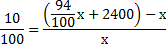10x=94x+240000-100x

4x=240000

16x=240000

X=15000

Profit%=(18000-15000)/15000×100=20%

Q.2) If A sells a table at 10% profit to B and B sells to C at 15% profit and C sells to D at 25% profit, then what is the cost price of A if cost price of D is Rs.5060.

a) Rs.4600

b) Rs.3200

c) Rs.4200

d) Rs.3600

e) None of these

b

Let cost price of A be x.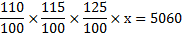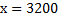Q.3) Akhil bought 20 kg of Ragi at the rate of Rs 21.50 per kg and 15 kg at the rate of Rs 18.50 per kg. He mixed the two and sold the mixture at the rate of Rs 20.50 per kg. What was his total gain in this transaction?

a) Rs.100

b) Rs.50

c) Rs.20

d) Rs.40

e) Rs.10

e

Total cost price=(20×21.50)+(15×18.50)=430+277.5=707.5

Total selling price=35×20.50=717.5

Gain=717.5-707.5=Rs.10

Q.4) Ajay bought 2 old air conditioners for Rs.50000. He spent total amount of Rs.25000 for the maintenance and repairing of these two air conditioners. If he sold one air conditioner for Rs.45000, then what is the selling price of another air conditioner to make 15% profit in total?

a) Rs.39500

b) Rs.41250

c) Rs.43250

d) Rs.40750

e) None of these

b

Total cost price of two AC=50000+25000=75000

Required selling price=75000×115/100-45000=86250-45000=RS.41250

Q.5) Cost of 5 kg of carrot is equal to the cost of 3 kg of tomato, cost of 5 kg of tomato is equal to the cost of 2kg of brinjal, and cost of 4 kg of brinjal is equal to the cost of 5 kg of potato. If 1 kg of potato is Rs.20, then what is the cost of 3 kg of carrot?

a) Rs.25

b) Rs.12

c) Rs.18

d) Rs.32

e) None of these

c

5 kg carrot=3 kg tomato

5 kg tomato=2 kg brinjal

4 kg brinjal =5 kg potato=100

5 kg tomato=100/4×2=50

5kg carrot=50/5×3=30

1 kg carrot=30/5=6

3 kg carrot=3×6=Rs.18

Q.6) If the difference between the selling prices of an article at a profit of 10% and 15% is Rs.36, then what is the sum of the two selling prices (In Rs)?

a) 1440

b) 1620

c) 1580

d) 1720

e) 1660

b

Let the cost price be x.

1.15x-1.10x=36

x=36/0.05=720

Required sum=1.15×720+1.10×720=828+792=1620

Q.7) A shopkeeper marks the price of the product by 45% above the cost price and then offers two successive discounts of 12% and 10% respectively. What is percentage loss and percentage gain? (Approximately)

a) 15% loss

b) 20% loss

c) 20% gain

d) 15% gain

e) None of these

d

Let cost price of the product be x.

Marked price=1.45 x

Selling price=1.45x×88/100×90/100=11484/10000

%Profit=(11484-10000)/10000×100= 14.84%~15%

Q.8) While selling a land, the profit is 150% of the cost price. If the cost increases by 35% but the selling price remains constant, then what is the profit percentage? (Approximately)

a) 90%

b) 95%

c) 85%

d) 80%

e) None of these

c

Let the cost price be Rs.100.

Profit=150

Selling price=150+100=250

If cost is increased to 35%, profit percentage=(250-135)/135×100=85% (Approximately)

Q.9) If the cost price of an article triples then the percentage loss doubles. What is the initial loss %?

a) 80%

b) 60%

c) 40%

d) 75%

e) 70%

c

Let the cost price be C.P and selling price be S.P.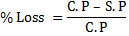When the cost price triples, the loss gets double.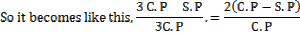3C.P-S.P=6 C.P-6S.P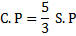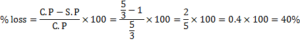Q.10) Raja invests certain amounts in two schemes A and B in the ratio of 3:8 and earns 20% on scheme A but loses 15% on scheme B. What is the gain or loss on two investments taken together?

a) 6.25%

b) 4.28%

c) 5.45%

d) Cannot be determined

e) None of these

c

Total investment=3x+8x=11x

gain in scheme A=0.2×3x=0.6x

Loss in scheme B=0.15×8=1.2x

Total loss=0.6 x

% loss=0.6/11×100=5.45%